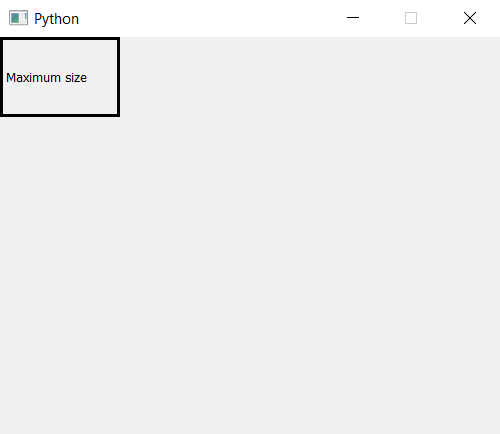Open in App
Not now

# PyQt5 – Set maximum window size

• Last Updated : 26 Mar, 2020

When we create a window, by default, it is resizable but with the help of `setFixedSize()` method we can fix the size of window. But what if we want to resize the window up-to some extent; in order to do this, we have to set the maximum size of the window. We will use `setMaximumSize()` method.

Syntax : self.setMaximumSize(width, height)

Argument : It takes two argument both integer i.e width and height.

Action performed : It set the maximum size of window.

Code :

 `# importing the required libraries`` ` `from` `PyQt5.QtCore ``import` `*` `from` `PyQt5.QtGui ``import` `*` `from` `PyQt5.QtWidgets ``import` `*` `import` `sys`` ` ` ` `class` `Window(QMainWindow):``    ``def` `__init__(``self``):``        ``super``().__init__()`` ` ` ` `        ``# set the title``        ``self``.setWindowTitle(``"Python"``)`` ` `        ``width ``=` `500``        ``height ``=` `400``        ``# setting the maximum size``        ``self``.setMaximumSize(width, height)`` ` ` ` `        ``# creating a label widget``        ``self``.label_1 ``=` `QLabel(``"Maximum size"``, ``self``)`` ` `        ``# moving position``        ``self``.label_1.move(``0``, ``0``)`` ` `        ``# setting up the border``        ``self``.label_1.setStyleSheet(``"border :3px solid black;"``)`` ` `        ``# resizing label``        ``self``.label_1.resize(``120``, ``80``)`` ` `        ``# show all the widgets``        ``self``.show()`` ` ` ` `# create pyqt5 app``App ``=` `QApplication(sys.argv)`` ` `# create the instance of our Window``window ``=` `Window()`` ` `# start the app``sys.exit(App.``exec``())`

Output :My Personal Notes arrow_drop_up